cancel
Showing results for
Did you mean:

## Use Incorta Dashboard for Confusion MatrixEmployee

In this article, you will learn how to create the Confusion Matrix with Incorta Dashboard. Confusion Matrix is a table that shows the performance in machine learning classification. Confusion Matrix includes Accuracy, Precision, Recall, and F1. At this point, you will calculate Accuracy, Precision Rate, and Recall Rate in Incorta Dashboard.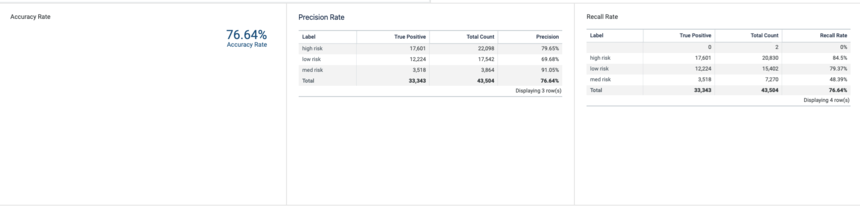### Accuracy

Accuracy is calculated from all the classes(positive and negative) to show how many of output predicted correctly. Accuracy should be high as possible.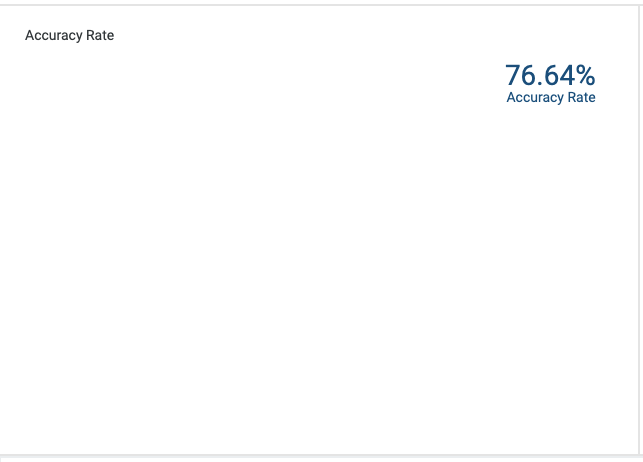In the Insight, Select KPI. Add Formula to Measure, below code:

``````sum(
if(
SCHEMANAME.TABLENAME.LABEL = SCHEMANAME.TABLENAME.PREDICTEDLABEL,
1,
0
)
) /
count(
SCHEMANAME.TABLENAME.LABEL
)``````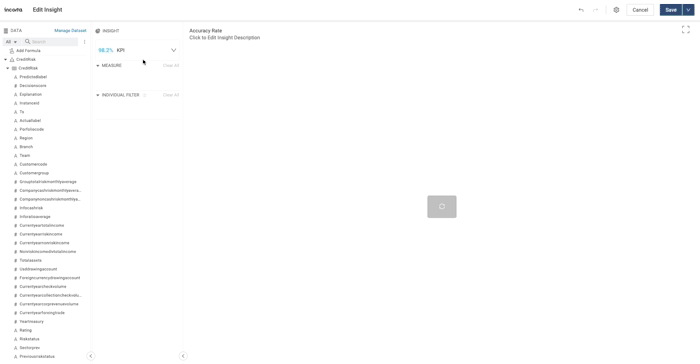### Precision Rate

Precision Rate is calculated for each class you have predicted and predicted accurately. Precision should be high as possible.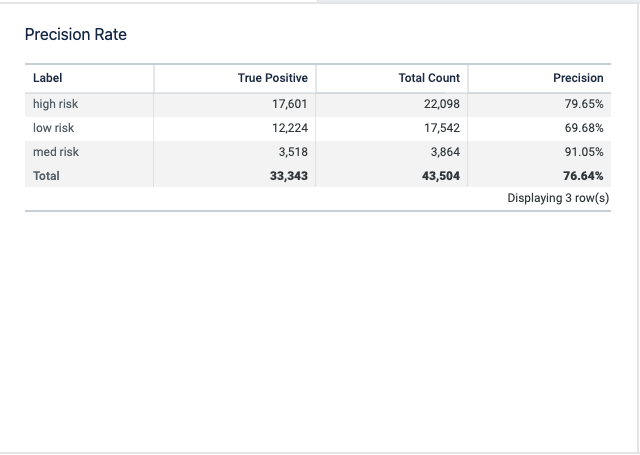In the Insight, Select Aggregated Table. Drag and drop the PredictedLabel column to Grouping Dimension. Add Formula named: True Positive to Measure, below codes:

``````sum(
if(
SCHEMANAME.TABLENAME.PREDICTEDLABEL = SCHEMANAME.TABLENAME.LABEL,
1,
0
)
)``````

Drag and drop the PredictedLabel column to MeasureAdd Formula named: Precision to Measure, following below codes:

``````sum(
if(
SCHEMANAME.TABLENAME.PREDICTEDLABEL = SCHEMANAME.TABLENAME.LABEL,
1,
0
)
) / count(
SCHEMANAME.TABLENAME.PREDICTEDLABEL
)``````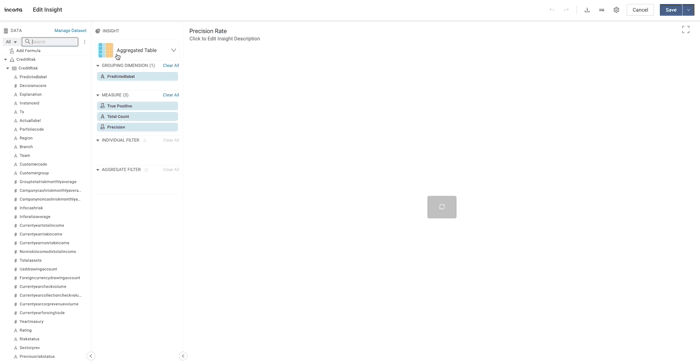### Recall Rate

Recall Rate is calculated for each class you have from the sample and predicted accurately. Precision should be high as possible.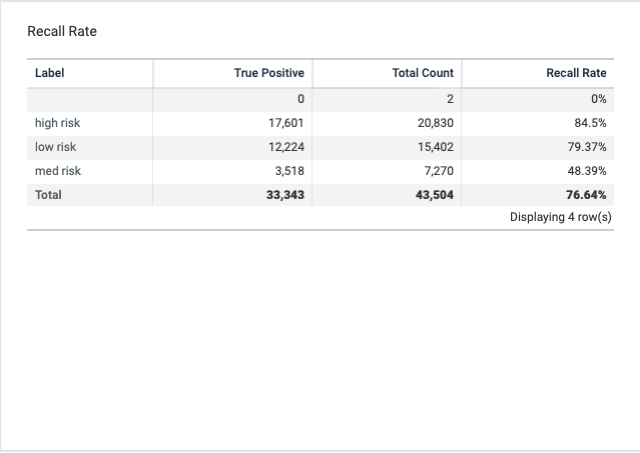In the Insight, Select Aggregated Table. Drag and drop the Label column to Grouping Dimension. Add Formula named: True Positive to Measure, following below codes:

``````sum(
if(
SCHEMANAME.TABLENAME.PREDICTEDLABEL = SCHEMANAME.TABLENAME.LABEL,
1,
0
)
)``````

Drag and drop the PredictedLabel column to measureAdd Formula named: Recall to Measure, following below codes:

``````sum(
if(
SCHEMANAME.TABLENAME.PREDICTEDLABEL = SCHEMANAME.TABLENAME.LABEL,
1,
0
)
) / count(
SCHEMANAME.TABLENAME.PREDICTEDLABEL
)``````
Best Practices IndexJust here to browse knowledge? This might help!

Contributors
Version history
Last update:
‎03-08-2022 03:12 PM
Updated by: